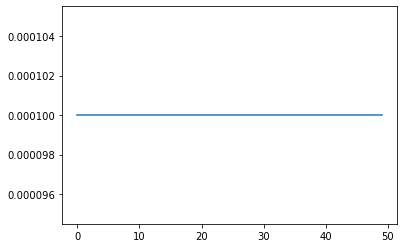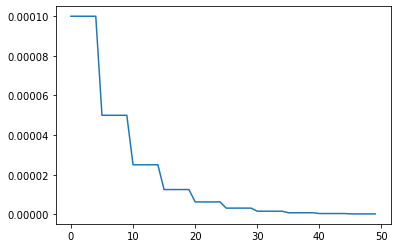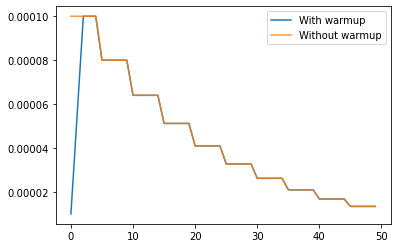In this tutorial we are going to be looking at the StepLRScheduler in the timm library.

The schedule looks something like:

from timm.scheduler.step_lr import StepLRScheduler
from nbdev.showdoc import show_doc


## classStepLRScheduler[source]

StepLRScheduler(optimizer:Optimizer, decay_t:float, decay_rate:float=1.0, warmup_t=0, warmup_lr_init=0, t_in_epochs=True, noise_range_t=None, noise_pct=0.67, noise_std=1.0, noise_seed=42, initialize=True) :: Scheduler

The StepLRScheduler as shown above accepts an optimizer and also some hyperparams which we will look into in detail below. We will first see how we can train models using the StepLRScheduler by first using timm training docs and then look at how we can use this scheduler as standalone scheduler for our custom training scripts.

## Using StepLRScheduler scheduler with timm training script

To train models using the StepLRScheduler we simply update the training script args passed by passing in --sched step parameter alongside the necessary hyperparams. In this section we will also look at how each of the hyperparams update the cosine scheduler.

The training command to use cosine scheduler looks something like:

python train.py ../imagenette2-320/ --sched step


## Args

This way we start to use the StepLRScheduler with all the defaults. Let's now look at the associated hyperparams and how that updates the annealing schedule.

### optimizer

This is the optimizer that will be used for the training process.

from timm import create_model
from timm.optim import create_optimizer
from types import SimpleNamespace

model = create_model('resnet34')

args = SimpleNamespace()
args.weight_decay = 0
args.lr = 1e-4
args.momentum = 0.9

optimizer = create_optimizer(args, model)


This optimizer object created using create_optimizer is what get's passed to the optimizer argument.

### decay_t

The number of epochs after which to decay the learning rate where the new learning rate value equals lr * decay_rate.

### decay_rate

When decay_rate > 0 and <1., at every restart the learning rate is decayed by new learning rate which equals lr * decay_rate. So if decay_rate=0.5, then in that case, the new learning rate becomes half the initial lr.

from matplotlib import pyplot as plt

def get_lr_per_epoch(scheduler, num_epoch):
lr_per_epoch = []
for epoch in range(num_epoch):
lr_per_epoch.append(scheduler.get_epoch_values(epoch))
return lr_per_epoch


#### decay_rate=1. or no decay

By setting decay_t = 5 and decay_rate = 1., we are telling the schedule to reduce the learning rate by decay_rate where new lr lr * decay_rate every 5 epochs.

But since, decay_rate=1., the new learning rate equals the old learning rate hence, we get a constant line.

num_epoch = 50
scheduler = StepLRScheduler(optimizer, decay_t = 5, decay_rate=1.)
lr_per_epoch = get_lr_per_epoch(scheduler, num_epoch)

plt.plot([i for i in range(num_epoch)], lr_per_epoch);#### decay_rate=0.5

By setting decay_t = 5 and decay_rate = 0.5, we are telling the schedule to reduce the learning rate by decay_rate where new lr lr * decay_rate every 5 epochs, that is halve the learning rate after every 5 epochs.

num_epoch = 50
scheduler = StepLRScheduler(optimizer, decay_t = 5, decay_rate=.5)
lr_per_epoch = get_lr_per_epoch(scheduler, num_epoch)

plt.plot([i for i in range(num_epoch)], lr_per_epoch);### warmup_t

Defines the number of warmup epochs.

### warmup_lr_init

The initial learning rate during warmup.

num_epoch = 50
scheduler = StepLRScheduler(optimizer, decay_t=5, warmup_t=2, warmup_lr_init=1e-5, decay_rate=0.8)
lr_per_epoch = get_lr_per_epoch(scheduler, num_epoch)
plt.plot([i for i in range(num_epoch)], lr_per_epoch, label="With warmup");

num_epoch = 50
scheduler = StepLRScheduler(optimizer, decay_t=5, decay_rate=0.8)
lr_per_epoch = get_lr_per_epoch(scheduler, num_epoch)
plt.plot([i for i in range(num_epoch)], lr_per_epoch, label="Without warmup", alpha=0.8);

plt.legend();As we can see by setting up warmup_t and warmup_lr_init, the cosine scheduler first starts with a value of warmup_lr_init, then gradually progresses up to the initial_lr set in the optimizer which is 1e-4. It takes warmup_t number of epochs to go from warmup_lr_init to initial_lr.

### noise_range_t

The upper and lower limit of noise.

### noise_pct

Percentage of noise to add.

### noise_std

Noise standard deviation.

### noise_seed

Seed to use to add random noise.

### t_in_epochs

If set to False, the learning rates returned for epoch t are None.

num_epoch = 50
scheduler = StepLRScheduler(optimizer, decay_t=5, t_in_epochs=False)
lr_per_epoch = get_lr_per_epoch(scheduler, num_epoch)

lr_per_epoch[:5]

[None, None, None, None, None]

### initialize

If True, then inside each param group of the optimizer a new field is set called initial_{field_name} where field_name refers to the field in param group that we are scheduling. Typically field_name='lr'.